### IMO Shortlist 2002 problem C6

Kvaliteta:
Avg: 0,0
Težina:
Avg: 8,0
Let$n$ be an even positive integer. Show that there is a permutation$\left(x_{1},x_{2},\ldots,x_{n}\right)$ of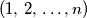$\left(1,\,2,\,\ldots,n\right)$ such that for every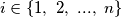$i\in\left\{1,\ 2,\ ...,\ n\right\}$, the number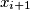$x_{i+1}$ is one of the numbers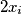$2x_{i}$,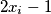$2x_{i}-1$,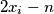$2x_{i}-n$,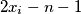$2x_{i}-n-1$. Hereby, we use the cyclic subscript convention, so that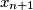$x_{n+1}$ means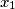$x_{1}$.
Izvor: Međunarodna matematička olimpijada, shortlist 2002